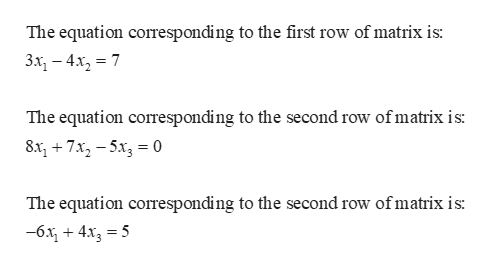# 11 of 23 (5 complete)This Test: 23 pts pWrite the system of linear equations that is represented by the following augmented matrix. Assume that the variables are x, x2, ...0 73 -47 -5 04 5-60What equation corresponds to the first row of the matrix?(Type your answer in standard form.)What equation corresponds to the second row of the matrix?(Type your answer in standard form.)HWhat equation corresponds to the third row of the matrix?(Type your answer in standard form.)k QEnter your answer in each of the answer boxes.Kck Qeck Homework

Question
check_circleExpert Solution

The equations are g...help_outlineImage TranscriptioncloseThe equation corresponding to the first row of matrix is: 3x, — 4х, %3D 7 The equation corresponding to the second row of matrix is 83+7x2-5x 0 The equation corresponding to the second row ofmatrix is -6х, + 4х, — 5 fullscreen

### Want to see the full answer?

See Solution

#### Want to see this answer and more?

Solutions are written by subject experts who are available 24/7. Questions are typically answered within 1 hour*

See Solution
*Response times may vary by subject and question
Tagged in

### Algebra